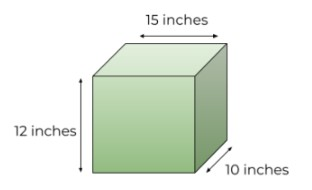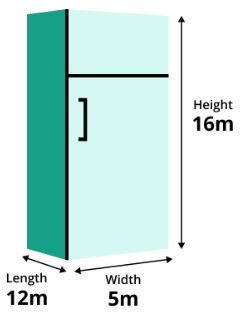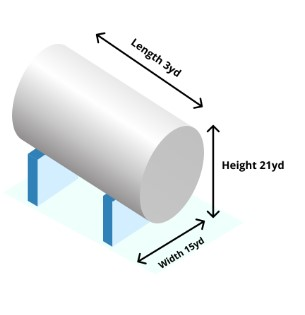# Cubic Feet Calculator

To calculate cubic feet enter length, height, and width in required input boxes, select unit, and press calculate button using cubic feet calculator

Formula:
Cubic Feet = Length (ft) × Width (ft) × Height (ft)

Give Us Feedback

## Cubic Feet Calcualtor

Convert the cubic inches to feet using the cubic feet calculator. This cubic ft calculator will provide the conversion from inches, yards, meters, centimeters, and millimeters to feet for each measurement and the overall conversion as well.

You will also find the visual representation of the cube for the required measurement. This gives an idea about the geometrical representation of the object.

## What is a cubic foot?

A cube is something present in three dimensions whereas a square is always two-dimensional. It has 3 measurements i.e. Length, Width, and Height. A foot is a unit of length just like inches or yards. So, a cubic foot makes a unit of volume, the one that is calculated in feet.

The volume of a cube is also presented in other units like cubic meters and cubic yards. Each has its own importance. A cubic foot is required at the industrial level for shipping and construction purposes.

## Cubic foot formula from meters, inches, and yards:

Cubic foot shares the same formula as volume. This formula is:

`Volume = length x width x height`

Here are the methods to convert other units in feet.

### Meters to feet

To change the unit from `meters to feet`, multiply each value by 3.28. But if the volume is already calculated, then multiply the result by 35.31.

### Inches to feet

To change the unit from `inches to feet`, divide each value by 12. But if the volume is already calculated, then divide the whole value by 1728.

### Yards to feet

To change the unit from `yards to feet`, multiply each value by 3. But if the volume is already calculated, then multiply the whole value by 27.

## How to calculate the cubic feet from meters, inches, and yards?

We recommend using the Cubic feet calculator to find the volume from measurements in different units.

To find it yourself, you have a choice between two methods. First is:

1. Find the volume of the cube using the mentioned formula.
2. Convert the volume from cubic inches to cubic feet.

The second method is.

1. Convert each length separately from inches to feet.
2. Multiply them.
3. The answer is in cubic feet.

### Example: From inches to feet

Find the volume in the cubic foot for the following object.Solution:

Step 1:  Write the measurements.

Length = 15 inches

Width = 10 inches

Height = 12 inches

Step 2: Convert the inches into feet.

Length = 15 / 12 = 1.25 ft

Width = 10 / 12 = 0.83 ft

Height = 12 / 12 = 1 ft

Step 3: Find the volume using its formula.

= L x W x H

= 1.25 x 0.83 x 1

= 1.0375 ft3

### Example 2: From meters to feet

Calculate cubic feet of the given measurements of a refrigerator.Solution

Step 1:  Write the measurements of a refrigerator.

Length = 12m

Width = 5m

Height = 16m

Step 2: Convert the meters into feet.

Length = 12 x 3.28 = 39.36 ft

Width = 5 x 3.28 = 16.4 ft

Height = 16 x 3.28 = 52.48 ft

Step 3: Find the volume using its formula.

= L x W x H

= 39.36 x 16.4 x 52.48

= 33876.05 ft3

### Example 3: From yards to feet

Calculate cubic feet of the given measurements of a cylindrical tank.Solution

Step 1:  Write the measurements of a cylindrical tank.

Length = 3yd

Width = 15yd

Height = 21yd

Step 2: Convert the yards into feet.

Length = 3 x 3 = 9 ft

Width = 15 x 3 = 45 ft

Height = 21 x 3 = 63 ft

Step 3: Find the volume using its formula.

= L x W x H

= 9 x 45 x 63

= 25515 ft3

## FAQs

How to calculate cubic feet from inches?

Calculate the volume of the measurements according to the inches and after that divide the result by 1728. Or convert each measurement from inches to feet by dividing each value by 12.

How do you measure cubic feet?

Cubic feet can be measured by multiplying all the measurements after converting them into feet.

### Math Tools